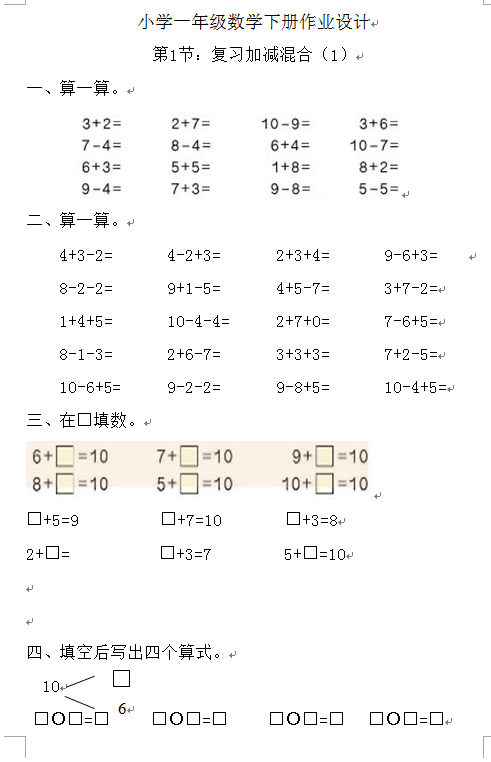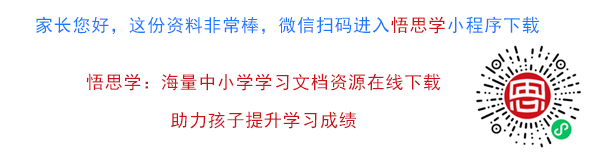1. 复习加减混合（1）
2. 算一算。

4+3-2=       4-2+3=       2+3+4=       9-6+3=

8-2-2=       9+1-5=       4+5-7=       3+7-2=

1+4+5=       10-4-4=      2+7+0=       7-6+5=

【DOC文档77页】小学一年级下册数学练习题全套A4电子版资料_可直接打印_会员免费下载##### 评论信息